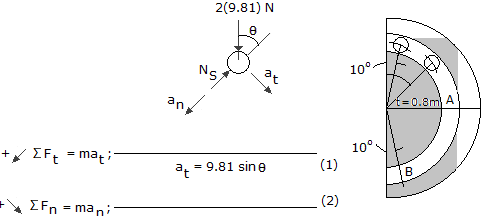# Engineering Mechanics - KOP: Force and Acceleration - Discussion

### Discussion :: KOP: Force and Acceleration - General Questions (Q.No.5)

5.A ball having a mass of 2 kg slides without friction within a vertical circular slot. If it is released from rest when= 10°, determine the force it exerts on the slot when it arrives at points A and B.

 [A]. NA = 38.6 N, NB = 96.6 N [B]. NA = 30.9 N, NB = 61.8 N [C]. NA = 30.9 N, NB = 81.2 N [D]. NA = 38.6 N, NB = 77.3 N

Explanation:

No answer description available for this question.